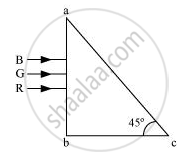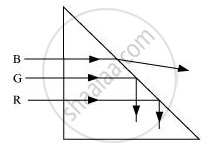Advertisement Remove all ads

# Three Light Rays Red (R), Green (G) and Blue (B) Are Incident on a Right Angled Prism ‘Abc’ at Face ‘Ab’. the Refractive Indices of the Material of the Prism for Red, Green and Blue Wavelengths Are - Physics

Advertisement Remove all ads
Advertisement Remove all ads
Advertisement Remove all ads

Three light rays red (R), green (G) and blue (B) are incident on a right angled prism ‘abc’ at face ‘ab’. The refractive indices of the material of the prism for red, green and blue wavelengths are 1.39, 1.44 and 1.47 respectively. Out of the three which colour ray will emerge out of face ‘ac’? Justify your answer. Trace the path of these rays after passing through face ‘ab’.Advertisement Remove all ads

#### SolutionThe blue ray will emerge out of the face ‘ac’.

The three light rays will go through ‘ab’ as the three are perpendicular to ‘ab’. However, when they will hit ‘ac’, they will refract with an angle of incidence of 45°.

Refractive indices for three light rays are given.

Red (R) = 1.39

Green (G) = 1.44

Blue (B) = 1.47

Total internal reflection takes place if the angle of incidence is such that

sin r>I

The refractive indices are from air to prism. To convert them from prism to air, we take their reciprocal.

R = 1/(1.39) = mu_1

G = 1/(1.44) =mu_2

B1/(1.47) =mu_3

For red:

(sini)/(sinr) =mu

(sin45)/mu_1  = sinr

sin r = 0.9828, which is less than 1

Thus, red light will pass through the face ‘ac’.

Similarly, for green:

sinr = (sin45)/mu_2

1.01

This is greater than 1. Therefore, it will not pass through, but reflect back in the same medium.

For blue:

sinr = (sin45)/mu_3

= 1.039

Even this will reflect back because of total internal reflection.

Concept: Refraction Through a Prism
Is there an error in this question or solution?

#### APPEARS IN

Advertisement Remove all ads
Share
Notifications

View all notifications

Forgot password?